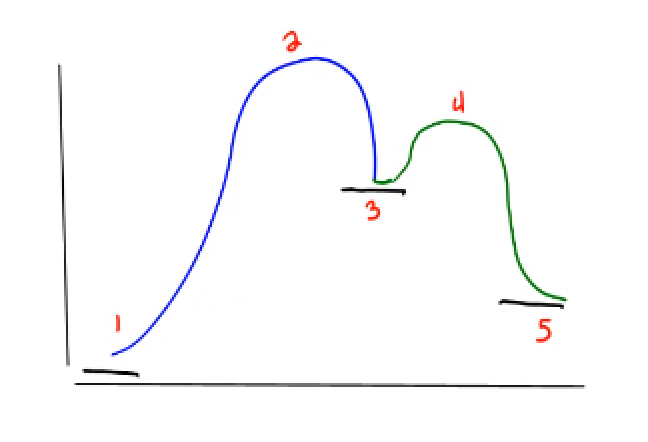Problem: According to the diagram, the activation energy of the slow step is given by the energy difference between statesA. 1 and 2B. 2 and 3C. 3 and 4D. 1 and 5

FREE Expert Solution
81% (114 ratings)
Problem Details

According to the diagram, the activation energy of the slow step is given by the energy difference between statesA. 1 and 2

B. 2 and 3

C. 3 and 4

D. 1 and 5